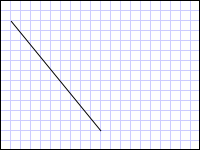# How to: Create a Line Using a LineGeometry

This example shows how to use the LineGeometry class to describe a line. A LineGeometry is defined by its start and end points.

## Example

The following example shows how to create and render a LineGeometry. A Path element is used to render the line. Since a line has no area, the Path object's Fill is not specified; instead the Stroke and StrokeThickness properties are used.

``````<Path Stroke="Black" StrokeThickness="1" >
<Path.Data>
<LineGeometry StartPoint="10,20" EndPoint="100,130" />
</Path.Data>
</Path>
``````
``````LineGeometry myLineGeometry = new LineGeometry();
myLineGeometry.StartPoint = new Point(10,20);
myLineGeometry.EndPoint = new Point(100,130);

Path myPath = new Path();
myPath.Stroke = Brushes.Black;
myPath.StrokeThickness = 1;
myPath.Data = myLineGeometry;
``````
``````Dim myLineGeometry As New LineGeometry()
myLineGeometry.StartPoint = New Point(10,20)
myLineGeometry.EndPoint = New Point(100,130)

Dim myPath As New Path()
myPath.Stroke = Brushes.Black
myPath.StrokeThickness = 1
myPath.Data = myLineGeometry
``````A LineGeometry drawn from (10,20) to (100,130)

Other simple geometry classes include LineGeometry and EllipseGeometry. These geometries, as well as more complex ones, can also be created using a PathGeometry or StreamGeometry. For more information, see the Geometry Overview.